• 周期性连续信号的频谱
千次阅读
2020-12-21 18:41:31

对于初学数字信号处理(DSP)的人来说，这几种变换是最为头疼的，它们是数字信号处理的理论基础，贯穿整个信号的处理。

学习过《高等数学》和《信号与系统》这两门课的朋友，都知道时域上任意连续的周期信号可以分解为无限多个正弦信号之和，在频域上就表示为离散非周期的信号，即时域连续周期对应频域离散非周期的特点，这就是傅里叶级数展开(FS)，它用于分析连续周期信号。

FT是傅里叶变换，它主要用于分析连续非周期信号，由于信号是非周期的，它必包含了各种频率的信号，所以具有时域连续非周期对应频域连续非周期的特点。

FS和FT 都是用于连续信号频谱的分析工具，它们都以傅里叶级数理论问基础推导出的。时域上连续的信号在频域上都有非周期的特点，但对于周期信号和非周期信号又有在频域离散和连续之分。

在自然界中除了存在温度，压力等在时间上连续的信号，还存在一些离散信号，离散信号可经过连续信号采样获得，也有本身就是离散的。例如，某地区的年降水量 或平均增长率等信号，这类信号的时间变量为年，不在整数时间点的信号是没有意义的。用于离散信号频谱分析的工具包括DFS，DTFT和DFT。

DTFT是离散时间傅里叶变换 ，它用于离散非周期序列分析，根据连续傅里叶变换要求连续信号在时间上必须可积这一充分必要条件，那么对于离散时间傅里叶变换，用于它之上的离散序列也必 须满足在时间轴上级数求和收敛的条件；由于信号是非周期序列，它必包含了各种频率的信号，所以DTFT对离散非周期信号变换后的频谱为连续的，即有时域离 散非周期对应频域连续周期的特点。

当离散的信号为周期序列时，严格的讲，离散时间傅里叶变换是不存在的，因为它不满足信号序列绝对级数和收敛(绝对可和)这一傅里叶变换的充要条件，但是采用DFS(离散傅里叶级数)这一分析工具仍然可以对其进行傅里叶分析。

我们知道周期离散信号是由无穷多相同的周期序列在时间轴上组成的，假设周期为N，即每个周期序列都有N个元素，而这样的周期序列有无穷多个，由于无穷多个 周期序列都相同，所以可以只取其中一个周期就足以表示整个序列了，这个被抽出来表示整个序列特性的周期称为主值周期，这个序列称为主值序列。然后以N对应 的频率作为基频构成傅里叶级数展开所需要的复指数序列ek(n)=exp(j*2pi*k*n/N)，用主值序列与复指数序列取相关(乘加运算)，得出每 个主值在各频率上的频谱分量，这样就表示出了周期序列的频谱特性。

根据DTFT，对于有限长序列作Z变换或序列傅里叶变换都是可行的，或者说，有限长序列的频域和复频域分析在理论上都已经解决；但对于数字系统，无论是Z 变换还是序列傅里叶变换的适用方面都存在一些问题，重要是因为频率变量的连续性性质(DTFT变换出连续频谱)，不便于数字运算和储存。

参考DFS，可以采用类似DFS的分析方法对解决以上问题。可以把有限长非周期序列假设为一无限长周期序列的一个主直周期，即对有限长非周期序列进行周期 延拓，延拓后的序列完全可以采用DFS进行处理，即采用复指数基频序列和此有限长时间序列取相关，得出每个主值在各频率上的频谱分量以表示出这个“主值周 期”的频谱信息。

由于DFT借用了DFS，这样就假设了序列的周期无限性，但在处理时又对区间作出限定(主值区间)，以符合有限长的特点，这就使DFT带有了周期性。另 外，DFT只是对一周期内的有限个离散频率的表示，所以它在频率上是离散的，就相当于DTFT变换成连续频谱后再对其采样，此时采样频率等于序列延拓后的 周期N，即主值序列的个数。

下面谈谈DFS，DTFT，DFT，FFT的联系与区别

DFT与FFT其实是一个本质，FFT是DFT的一种快速算法。

DFS是discrete fourier seriers，对离散周期信号进行级数展开。DFT是将DFS取主值，DFS是DFT的周期延拓。

DTFT是对Discrete time fourier transformation，是对序列的FT，得到连续的周期谱，而DFT，FFT得到是有限长的非周期离散谱，不是一个。

DTFT与DFT的关系

我们知道，一个N点离散时间序列的傅里叶变换(DTFT)所的频谱是以(2*pi)为周期进行延拓的连续函数，由采样定理我们知道，时域进行采样，则频域 周期延拓；同理，如果在频域进行采样，则时域也会周期延拓。离散傅里叶变换(DFT)就是基于这个理论，在频域进行采样，一个周期内采N个点(与序列点数 相同) ，从而将信号的频谱离散化，得到一的重要的对应关系：一个N点离散时间信号可以用频域内一个N点序列来唯一确定，这就是DFT表达式所揭示的内容。

至于离散傅里叶变换DFT，其实也是对数字信号变换到频域进行分析处理，它对数字信号处理的作用相当大。数字信号处理脱离了模拟时期对信号进行处理完全依赖 于器件的情况，可以直接通过计算来进行信号处理。如数字滤波器，只是用系统的系数对进入的数字信号进行一定的计算，信号出系统后即得到处理后的数据在时域 上的表达。

离散傅里叶变换在理解上与连续信号的傅里叶变换不太相同，主要是离散信号的傅里叶变换涉及到周期延拓，以及圆周卷积等。

快速傅里叶变换FFT其实是一种对离散傅里叶变换的快速算法，它的出现解决了离散傅里叶变换的计算量极大、不实用的问题，使离散傅里叶变换的计算量降低了 一个或几个数量级，从而使离散傅里叶变换得到了广泛应用。另外，FFT的出现也解决了相当多的计算问题，使得其它计算也可以通过FFT来解决。

更多相关内容
• 周期性函数可以在傅里叶级数中展开，也就是说，如果给定了各个频率分量的幅度和相位，则可以确定原信号频谱信号的一种图形表示方法，它将各个频率分量上的系数关系用图形的方法表示出来，用来说明信号的特性。...

周期性函数可以在傅里叶级数中展开，也就是说，如果给定了各个频率分量的幅度和相位，则可以确定原信号。频谱是信号的一种图形表示方法，它将各个频率分量上的系数关系用图形的方法表示出来，用来说明信号的特性。并且后续可以给信号处理带来很多便利。

频谱图由两个部分组成：振幅频谱相位频谱

• 振幅频谱用来表示信号含有的各个频率分量的幅度，其横坐标为频率，纵坐标为各个对应频率分量的幅度；

• 相位频谱用来表示含有各个频率分量的相位。其横坐标为频率，纵坐标为各个对应频率分量的相位。

傅里叶级数分为实数形式的傅里叶级数以及复数形式的傅里叶级数，因此，傅里叶级数的频谱对应的也有两种形式。

本篇文章中所用到的图像来自于东南大学孟桥老师的课件，感谢老师对学生的耐心讲解。

## 1. 实数形式傅里叶级数对应的频谱

由实数形式傅里叶级数：
f ( t ) = a 0 2 + ∑ n = 1 + ∞ A n c o s ( n Ω t + φ n ) A n = a n 2 + b n 2 , φ n = − a r c t a n b n a n \begin{aligned} f(t)&=\frac{a_0}{2}+\sum_{n=1}^{+\infty}A_ncos(n\Omega t + \varphi_n) \\A_n &= \sqrt{a_n^2+b_n^2}, \\\varphi_n &= -arctan\frac{b_n}{a_n} \end{aligned}
其对应的频谱图示意图如下：由于这种形式的傅里叶级数中的级数 n n 一定大于等于，因此这种频谱的横坐标仅有正数，这种频谱也称为单边频谱

比如周期性方波的频谱，其傅里叶级数为：
f ( t ) = 4 π [ s i n ( Ω t ) + 1 3 s i n ( 3 Ω t ) + 1 5 s i n ( 5 Ω t ) + ⋯ + 1 n s i n ( n Ω t ) ] , n 为 奇 数 f(t) = \frac{4}{\pi}[sin(\Omega t)+\frac{1}{3}sin(3\Omega t)+\frac{1}{5}sin(5\Omega t)+\cdots + \frac{1}{n}sin(n\Omega t)], n为奇数
即：
a n = 2 T ∫ 0 T f ( t ) c o s ( n Ω t ) d t = 0 a_n=\frac{2}{T}\int_{0}^{T}f(t)cos(n\Omega t)dt=0

b n = 2 T ∫ 0 T f ( t ) s i n ( n Ω t ) d t = { 4 n π ,    n 为 奇 数 0 ,    n 为 偶 数 b_n=\frac{2}{T}\int_{0}^{T}f(t)sin(n\Omega t)dt=\left\{ \begin{aligned} &\frac{4}{n\pi}&, \space \space &n为奇数 \\&0&, \space \space &n为偶数 \end{aligned} \right.

所以：
A n = a n 2 + b n 2 = { 4 n π     当 n 为 奇 数 0     当 n 为 偶 数 A_n = \sqrt{a_n^2+b_n^2}=\left\{ \begin{aligned} &\frac{4}{n\pi}\space \space \space&当n为奇数 \\&0\space \space \space&当n为偶数 \\ \end{aligned} \right.

φ n = − a r c t a n b n a n = { − π 2     当 n 为 奇 数 0     当 n 为 偶 数 \varphi_n = -arctan\frac{b_n}{a_n}=\left\{ \begin{aligned} &-\frac{\pi}{2}\space \space \space&当n为奇数 \\&0\space \space \space&当n为偶数 \\ \end{aligned} \right.

由此可以做出其频谱：在频谱中，横坐标表示的谐波的次数，但是更常见的，横坐标使用各个正弦分量的实际频率来表示。

单边频谱的特点

1. 在横坐标为0的点上，即直流分量上，幅度频谱中表示的直流分量幅值是真实信号幅值的两倍。即：频谱上在 ω = 0 \omega=0 的点上信号的实际的直流分量幅值应该是频谱中显示的幅值的一半
2. 如果仅观察幅值随频率的变化趋势，则无考虑。

## 2. 复指数形式傅里叶级数对应的频谱

由复指数形式的傅里叶级数：
f ( t ) = ∑ n = − ∞ + ∞ [ C n ⋅ e j ( n Ω t ) ] f(t)=\sum_{n=-\infty}^{+\infty}[C_n\cdot e^{j(n\Omega t)}]
其中：
C n = ∫ t 1 t 2 f ( t ) ⋅ ( e j n Ω t ) ∗ ∫ t 1 t 2 ( e j n Ω t ) ( e j n Ω t ) ∗ = 1 T ∫ t 1 t 2 f ( t ) e − j n Ω t d t C_n=\frac{\int_{t_1}^{t_2}f(t)\cdot (e^{j{n\Omega t}})^*}{\int_{t_1}^{t_2}(e^{j{n\Omega t}})(e^{j{n\Omega t}})^*} = \frac{1}{T}\int_{t_1}^{t_2}f(t)e^{-jn\Omega t}dt
其对应的频谱的幅值和相位为：
幅 值 ： ∣ C n ∣ 相 位 ： a n g ( C n ) \begin{aligned} &幅值：|C_n| \\&相位：ang(C_n) \end{aligned}
由于这种形式的傅里叶级数中的 n n 存在正值，也存在幅值，因此这种形式的频谱在 n ≥ 0   和 n < 0 n\geq0 \space 和 n<0 都有意义，这种频谱也称为双边频谱

同样以周期性方波的频谱为例：
C n = − j b n 2 = { − j 2 n π     当 n 为 奇 数 0     当 n 为 偶 数 C_n=-j\frac{b_n}{2}=\left \{ \begin{aligned} &-j\frac{2}{n\pi} \space \space \space&当n为奇数 \\&0\space \space \space&当n为偶数 \end{aligned} \right.
其频谱如下：双边频谱的特点

1. 从频谱图中观察可知：对于周期方波这个实数信号而言，其幅值是偶对称，而相位是奇对称。这与在信号与系统（9） 的分析吻合。单边频谱无法体现这一点。
2. 通常来说，由于实数信号的幅度频谱具备对称性，因此只需要观察 n ≥ 0 n\geq0 部分即可，其幅值形状与单边频谱类似，但是幅值上是单边频谱显示幅值的一半，相位频谱与单边频谱相同。
3. 单边频谱在物理上容易理解，而双边频谱对于信号处理会有计算上的优势，所以双边频谱是重点。
4. 双边频谱在 ω = 0 \omega = 0 的点上的幅值，即直流信号，其大小直接表示了信号的直流分量的大小，不需要其他处理。这要比单边频谱更方便。

## 3. 周期信号频谱有什么特点？

1. 离散性：其线条不是连续的
2. 谐波性：线条仅仅出现在基波频率 Ω \Omega 的整数倍点上
3. 收敛性：实际信号的幅频特性总是随着频率趋向无穷大而趋向于零

## 4. 周期信号的时域特性如何对应到频域特性？

周期信号的时域特性也就是信号的周期、脉冲宽度、幅值等，如何反应到频域特性如幅值、相位、谱线间隔、收敛性等呢？由于上述周期性方波信号的傅里叶级数展开后，其频谱无法体现时域信号中的参数，因此这里引入新的例子：周期性方波脉冲，即：
f ( t ) = { A ,     − τ 2 + k T ≤ t ≤ τ 2 + k T 0 ,     其 他 f(t)=\left\{ \begin{aligned} A,\space \space \space & -\frac{\tau}{2}+kT \leq t\leq\frac{\tau}{2}+kT \\0, \space \space \space &其他 \end{aligned} \right.
其图像如下所示：其中 τ \tau 是脉冲的宽度，T是周期，幅值是A。

经过傅里叶级数展开后，其系数为：
C n = { 2 A n Ω T s i n ( n Ω τ 2 )     n ≠ 0 2 A τ T     n = 0 C_n=\left\{ \begin{aligned} &\frac{2A}{n\Omega T}sin(\frac{n\Omega \tau}{2}) \space\space\space &n\neq0 \\&\frac{2A\tau}{T}\space \space\space&n=0 \end{aligned} \right.
观察上式可知，系数 C n C_n 可以用抽样函数 n ≠ 0 n\neq0 n = 0 n=0 统一起来，即：
C n = { 2 A n Ω T s i n ( n Ω τ 2 )     n ≠ 0 2 A τ T     n = 0 } = A τ T S a ( n Ω τ 2 ) C_n= \left\{ \begin{aligned} &\frac{2A}{n\Omega T}sin(\frac{n\Omega \tau}{2}) \space\space\space &n\neq0 \\&\frac{2A\tau}{T}\space \space\space&n=0 \end{aligned} \right\} =\frac{A\tau}{T}Sa(\frac{n\Omega \tau}{2})
其中 S a ( x ) = s i n x x Sa(x)=\frac{sinx}{x} ，是抽样函数。

通过上式画出周期方波脉冲信号的频谱如下：下面将通过改变时域中信号参数，观察频谱的变化，进而得出结论

1. 改变周期方波信号的周期T，保持脉冲宽度，其频谱变化如下从上述频谱中可以观察出3点：

• 谱线密度随着周期T的增加而增加，即随着T的增大，相邻谱线之间的间距越小。这是因为频率 f = 1 T f=\frac{1}{T} ，且 Ω = 2 π T \Omega = \frac{2\pi}{T} 的原因。很显然当T增加时， n Ω n\Omega 将变小，因此谱线之间的间距变小。
• 谱线的幅值逐渐降低。观察 C n = A τ T S a ( n Ω τ 2 ) C_n=\frac{A\tau}{T}Sa(\frac{n\Omega \tau}{2}) 可知，当T增大时，其幅值 A τ T \frac{A\tau}{T} 将减小。
• 不论T增大还是减小，谱线的包络线形状上保持不变，因为Sa函数除幅值外没有变化。

因此可以得出结论：信号周期加大，对增幅的收敛性没有影响，但是会使谱线的密度加大。当T趋近于无穷大时，信号成为非周期信号，谱线幅值无穷小，谱线密度加大，谱线称为连续谱线

2. 改变周期方波信号的脉冲宽度 τ \tau ，保持周期不变，其频谱变化如下观察谱线可以看出：

• 随着脉冲宽度下降，由 C n = A τ T S a ( n Ω τ 2 ) C_n=\frac{A\tau}{T}Sa(\frac{n\Omega \tau}{2}) 可知，Sa函数的尺度扩大，即零点的出现逐渐向后推迟。
• 收敛性变差，这是因为Sa函数的尺度逐渐变大导致的。
• 谱线的间隔不变，因为Sa函数中自变量的$n\Omega$没有改变。

因此可以得出结论：信号的宽度变小，信号的收敛性变差，谱线的间隔不变，且信号的能量向高频扩散

#### 问题：什么是信号的频带？

由于信号在频谱上的收敛性，可以将信号分量主要集中的频率区间作为主要研究对象，用来研究信号的特性，这部分频率区间称为信号的频带

定义频带有很多种方式，如：

1. 以信号最大幅度的1/10为限，其它部分忽略不计。
2. 以信号振幅频谱中的第一个过零点为限，零点以外部分忽略不计。
3. 以包含信号总能量的90%处为限，其余部分忽略不计。

如果信号的边沿变化的越快，则信号的频带越宽，也就是说包含的交流分量在幅值上随着频率的增加收敛的更慢。

#### 问题：周期方波脉冲的幅值为什么出现了负值？

事实上，对于复指数形式的傅里叶级数的频谱而言，其幅值应该为 C n C_n 的绝对值，即 ∣ C n ∣ |C_n| 来表示。但是由复变函数相关的内容可知，虚数往往意味着旋转，通常在复平面上，纵轴为虚轴坐标，横轴为实数坐标。对于一个实数信号来说，其幅值为实数，其幅值对应的相位只可能出现在实数坐标上，即相位仅可能是 π \pi − π -\pi 。因此，对于实数信号而言，仅需关心相位的符号即可。而Sa函数中，正好体现了幅值分量对应的相位符号。因此，可以用一副频谱图，体现相位、相位大小以及幅值大小等信息，无需再对相位进行求解。

谢谢阅读，如有不当之处，请批评指正！

展开全文• 连续信号的频域分析 由信号正交分解的思想可知，由于三角函数集是完备正交函数集，任意信号都可以分解为三角函数表达形式，换言之，任意信号都可视为一系列正弦信号的组合，这些正弦信号的频率、相位等特性势必反映...

## 连续信号的频域分析

由信号正交分解的思想可知，由于三角函数集是完备正交函数集，任意信号都可以分解为三角函数表达形式，换言之，任意信号都可视为一系列正弦信号的组合，这些正弦信号的频率、相位等特性势必反映了原信号的性质，这样出现了用频率域的特性来描述时间域信号的方法，即信号的频域分析法。频率特性是信号的客观性质，如光线的颜色、声音的音调，比信号的时域特性更能反映信号的基本特性。

### 周期信号的频谱分析

周期信号：
x ( t ) = x ( t + m T ) m = 0 , ± 1 , ± 2 , ⋯ x(t)=x(t+mT) \quad m=0,\pm1,\pm2,\cdots
信号的角频率为$w=2\pi f$

（一）周期信号的傅里叶级数展开式

狄利赫里条件：

1. 函数 x ( t ) x(t) 在一个周期内绝对可积，即 ∫ − T 0 2 T 0 2 ∣ x ( t ) ∣ d t < ∞ \int_{-\frac{T_0}{2}}^{\frac{T_0}{2}}|x(t)|dt<\infty
2. 函数在一个周期内只有有限个不连续点，在这些点上函数取有限值
3. 函数在一个周期内只有有限个极大值和极小值

通常的周期信号都满足该条件。

一个周期为 T 0 = 2 π w 0 T_0=\frac{2\pi}{w_0} 的周期信号，只要满足狄利赫里（Dirichlet）条件，都可以分解成三角函数表达式，即
x ( t ) = a 0 2 + ∑ n = 1 ∞ ( a n c o s   n w 0 t + b n s i n   n w 0 t ) (1) x(t)=\frac{a_0}{2}+\sum_{n=1}^\infty(a_ncos\,nw_0t+b_nsin\,nw_0t) \tag{1}
上式的无穷级数称为三角傅里叶级数。式中， a n ( n = 0 , 1 , 2 , ⋯   ) 、 b n ( n = 0 , 1 , 2 , ⋯   ) a_n(n=0,1,2,\cdots)、b_n(n=0,1,2,\cdots) 为傅里叶系数。

为了求得傅里叶系数，可将式（1）两边在一个周期内（为简单起见 − T 0 2 ∼ T 0 2 -\frac{T_0}{2}\sim \frac{T_0}{2} ）对时间进行积分，则得
∫ − T 0 2 T 0 2 x ( t ) d t = ∫ − T 0 2 T 0 2 a 0 2 d t + 0 = 1 2 a 0 T 0 a 0 = 2 T 0 ∫ − T 0 2 T 0 2 x ( t ) d t ( 2 ) \int_{-\frac{T_0}{2}}^{\frac{T_0}{2}}x(t)dt=\int_{-\frac{T_0}{2}}^{\frac{T_0}{2}}\frac{a_0}{2}dt+0=\frac{1}{2}a_0T_0 \\ a_0=\frac{2}{T_0}\int_{-\frac{T_0}{2}}^{\frac{T_0}{2}}x(t)dt \quad\quad\quad (2)
同理，将式（1）两边乘以 c o s   n w 0 t cos\,nw_0t 后在一个周期内求积分，并利用三角函数集的正交特性，得
∫ − T 0 2 T 0 2 x ( t ) c o s   n w 0 t d t = ∫ − T 0 2 T 0 2 a n c o s   n w 0 t ⋅ c o s   n w 0 t d t = 1 2 a n T 0 a n = 2 T 0 ∫ − T 0 2 T 0 2 x ( t ) c o s   n w 0 t d t n = 1 , 2 , ⋯ ( 3 ) \int_{-\frac{T_0}{2}}^{\frac{T_0}{2}}x(t)cos\,nw_0tdt=\int_{-\frac{T_0}{2}}^{\frac{T_0}{2}}a_ncos\,nw_0t·cos\,nw_0tdt = \frac{1}{2}a_nT_0 \\ a_n=\frac{2}{T_0}\int_{-\frac{T_0}{2}}^{\frac{T_0}{2}}x(t)cos\,nw_0tdt \quad n=1,2,\cdots \quad \quad(3)
类似地，将式（1）两边乘以 s i n   w 0 t sin\,w_0t 后在一个周期内求积分，得
b n = 2 T 0 ∫ − T 0 2 T 0 2 x ( t ) s i n   n w 0 t d t n = 1 , 2 , ⋯ b_n=\frac{2}{T_0}\int_{-\frac{T_0}{2}}^{\frac{T_0}{2}}x(t)sin\,nw_0tdt \quad n=1,2,\cdots
显然式（2）可以合并到式（3）中，并且 a n a_n b n b_n 分别是n的偶函数和奇函数。将式（1）中的同频率项合并，得
x ( t ) = A 0 2 + ∑ n = 1 ∞ A n c o s ( n w 0 t + φ n ) (4) x(t)=\frac{A_0}{2}+\sum_{n=1}^\infty A_ncos(nw_0t+\varphi_n) \tag{4}
式中
A 0 = a 0 , A n = a n 2 + b n 2 , φ n = − a r c t a n b n a n (5) A_0=a_0, \quad A_n =\sqrt{a_n^2+b_n^2} ,\quad \varphi_n=-arctan\frac{b_n}{a_n} \quad \tag{5}
式（4）是三角傅里叶级数的另外一种形式，它表明一个周期信号可以分解为直流分量和一系列余弦或正弦形式的交流分量。由式（2）可进一步知道，其中的直流分量是信号在一个周期内的平均值。

信号的三角傅里叶级数形式具有比较明确的物理意义，但运算不方便。在连续信号的时域描述中知道，正弦型信号和复指数型信号具有同一性。而且复指数函数集 { e j n w 0 t } ( n = 0 , ± 1 , ± 2 , ⋯   ) \{e^{jnw_0t}\}(n=0,\pm 1,\pm 2,\cdots) t 0 , t 0 + 2 π w 0 t_0,t_0+\frac{2\pi}{w_0} 内是完备正交函数集，因此，可以得出傅里叶级数的指数形式。

式（4）可以写成
x ( t ) = A 0 2 + ∑ n = 1 ∞ A n 2 [ e j ( n w 0 t + φ n ) + e − j ( n w 0 t + φ n ) ] = A 0 2 + 1 2 ∑ n = 1 ∞ A n e j φ n e j n w 0 t + 1 2 ∑ n = 1 ∞ A n e − j φ n e − j n w 0 t x(t)=\frac{A_0}{2}+\sum_{n=1}^\infty\frac{A_n}{2}[e^{j(nw_0t+\varphi_n)}+e^{-j(nw_0t+\varphi_n)}] \\ =\frac{A_0}{2}+\frac{1}{2}\sum_{n=1}^\infty A_ne^{j\varphi_n}e^{jnw_0t}+\frac{1}{2}\sum_{n=1}^{\infty}A_ne^{-j\varphi_n}e^{-jnw_0t}
由式（5）可知， A n A_n 是n的偶函数， φ n \varphi_n 是n的奇函数，上式可写为
x ( t ) = A 0 2 + 1 2 ∑ n = 1 ∞ A n e j w n e j n w 0 t + 1 2 ∑ n = − 1 − ∞ A n e j φ n e j n w 0 t x(t)=\frac{A_0}{2}+\frac{1}{2}\sum_{n=1}^{\infty}A_ne^{jw_n}e^{jnw_0t}+\frac{1}{2}\sum_{n=-1}^{-\infty}A_ne^{j\varphi_n}e^{jnw_0t}
考虑到 φ 0 = 0 \varphi_0=0 A 0 A_0 可以表示为 A 0 e j w 0 e j n w 0 t A_0e^{jw_0}e^{jnw_0t} ，上式可进一步写为
x ( t ) = 1 2 ∑ n = − ∞ ∞ A n e j φ n e j n w 0 t = ∑ n = − ∞ ∞ X ( n w 0 ) e j n w 0 t x(t)=\frac{1}{2}\sum_{n=-\infty}^{\infty}A_ne^{j\varphi_n}e^{jnw_0t}=\sum_{n=-\infty}^{\infty}X(nw_0)e^{jnw_0t}
这是傅里叶级数的指数形式，其中复数量 X ( n w 0 ) = 1 2 A n e j φ n X(nw_0)=\frac{1}{2}A_ne^{j\varphi_n} 称为复傅里叶系数，是n（或 n w 0 nw_0 ）的函数，可求得如下
X ( n w 0 ) = 1 2 A n e j φ n = 1 2 [ A n c o s φ n + j A n s i n φ n ] = 1 2 ( a n − j b n ) = 1 T 0 ∫ − T 0 2 T 0 2 x ( t ) c o s   n w 0 t d t − j 1 T 0 ∫ − T 0 2 T 0 2 x ( t ) s i n   n w 0 t d t = 1 T 0 ∫ − T 0 2 T 0 2 x ( t ) e − j n w 0 t d t n = 0 , ± 1 , ± 2 , ⋯ ( 6 ) X(nw_0)=\frac{1}{2}A_ne^{j\varphi_n}=\frac{1}{2}[A_ncos\varphi_n+jA_nsin\varphi_n] = \frac{1}{2}(a_n-jb_n) \\ =\frac{1}{T_0}\int_{-\frac{T_0}{2}}^{\frac{T_0}{2}}x(t)cos\,nw_0tdt-j\frac{1}{T_0}\int_{-\frac{T_0}{2}}^{\frac{T_0}{2}}x(t)sin\,nw_0tdt \\ =\frac{1}{T_0}\int_{-\frac{T_0}{2}}^{\frac{T_0}{2}}x(t)e^{-jnw_0t}dt \quad n=0,\pm1,\pm 2,\cdots \quad \quad (6)
式中， A n c o s φ n = a n , A n s i n φ n = − b n A_ncos\varphi_n=a_n,A_nsin\varphi_n=-b_n ，很容易从式（5）看出。

例1：求下图所示的周期矩形脉冲信号的复指数形式傅里叶级数表示式。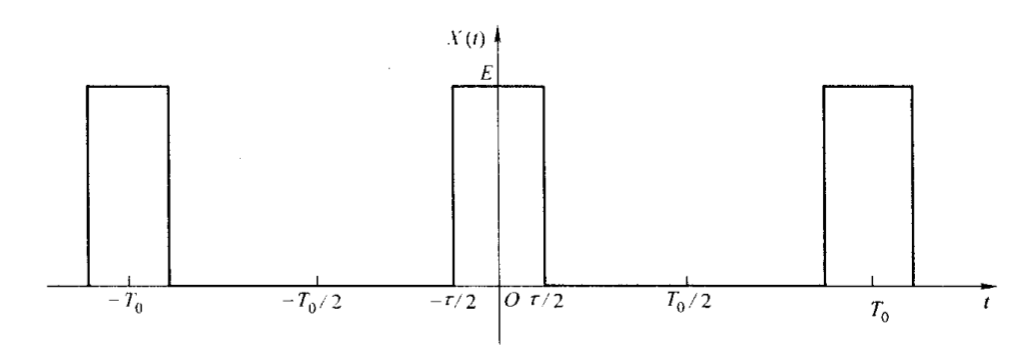矩形脉冲信号在一个周期内可表示为
x ( t ) = { E − τ 2 ≤ t ≤ τ 2 0 其 他 x(t)=\begin{cases} E & -\frac{\tau}{2}\leq t\leq \frac{\tau}{2} \\ 0 & 其他 \end{cases}
按式（6）可求得复指数形式傅里叶系数
X ( n w 0 ) = 1 T 0 ∫ − T 0 2 T 0 2 x ( t ) e − j n w 0 t d t = E τ T 0 s i n 1 2 n w 0 τ 1 2 n w 0 τ X(nw_0)=\frac{1}{T_0}\int_{-\frac{T_0}{2}}^{\frac{T_0}{2}}x(t)e^{-jnw_0t}dt=\frac{E\tau}{T_0}\frac{sin\frac{1}{2}nw_0\tau}{\frac{1}{2}nw_0\tau}

x ( t ) = E τ T 0 ∑ n = − ∞ ∞ S a ( n w 0 τ 2 ) e j n w 0 t x(t)=\frac{E\tau}{T_0}\sum_{n=-\infty}^{\infty}Sa(\frac{nw_0\tau}{2})e^{jnw_0t}

对于实信号 x ( t ) x(t) ，由于 X ∗ ( t ) = x ( t ) X^*(t)=x(t) ，有
x ( t ) = ∑ n = − ∞ ∞ X ∗ ( n w 0 ) e − j n w 0 t x(t)=\sum_{n=-\infty}^{\infty}X^*(nw_0)e^{-jnw_0t}
用-n代替n，则
x ( t ) = ∑ n = − ∞ ∞ X ∗ ( − n w 0 ) e j n w 0 t x(t)=\sum_{n=-\infty}^{\infty}X^*(-nw_0)e^{jnw_0t}
复傅里叶系数的重要性质： X ∗ ( − n w 0 ) = X ( n w 0 ) X^*(-nw_0)=X(nw_0) X ∗ ( n w 0 ) = X ( − n w 0 ) X^*(nw_0)=X(-nw_0)

取样函数 s i n x x \frac{sinx}{x} ，记作 S a ( x ) Sa(x) ，它是偶函数，当 x → 0 x\to 0 时， S a ( x ) = 1 Sa(x)=1 为最大值，随着 ∣ x ∣ |x| 的增大而总趋势衰减， x = ± π , ± 2 π , ⋯ x=\pm \pi,\pm 2\pi,\cdots 为过零点，每 2 π 2\pi 起伏一次，于是 X ( n w 0 ) X(nw_0) 可写成 X ( n w 0 ) = E τ T 0 S a ( n w 0 τ 2 ) , n = 0 , ± 1 , ⋯ X(nw_0)=\frac{E\tau}{T_0}Sa(\frac{nw_0\tau}{2}),\quad n=0,\pm1,\cdots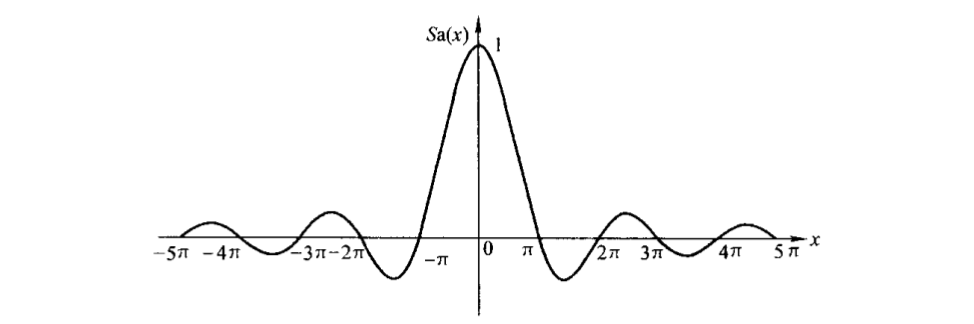可见例1所表示的周期矩形脉冲信号的复傅里叶系数是在 S a ( w τ 2 ) Sa(\frac{w\tau}{2}) 包络函数上以 w 0 w_0 等间隔取得的样本，其最大值 ( n = 0 处 ) (n=0处) 和过零点都由占空比 τ T 0 \frac{\tau}{T_0} 决定。

（二）周期信号的频谱

周期信号可以分解为一系列正弦型信号之和，即
x ( t ) = A 0 2 + ∑ n = 1 ∞ A n c o s ( n w 0 t + φ n ) x(t)=\frac{A_0}{2}+\sum_{n=1}^{\infty}A_ncos(nw_0t+\varphi_n)
它表明一个周期为 T 0 = 2 π w 0 T_0=\frac{2\pi}{w_0} 的信号，除直流分量（信号在一个周期内的平均值）外，包含了频率为原信号频率以及原信号频率的整数倍的一系列正弦型信号，分别将它们称为基波信号 ( n = 1 ) (n=1) （也称为，一次谐波信号）、二次谐波信号 ( n = 2 ) (n=2) ，以及三次、四次···谐波信号，它们的振幅分别为对应的 A n A_n ，相位分别为对应的 φ n \varphi_n 。可见周期信号的傅里叶级数展开式全面地描述了组成原信号的各正弦分量的特征：各谐波分量的频率、幅度和相位。因此也就等于全面地描述了原信号 x ( t ) x(t) 本身。换言之，对于一个周期信号，只要了信号的基频 w 0 w_0 、各谐波的幅度 A n A_n 和相位 φ n \varphi_n ，就等于掌握了该信号的所有特征。

指数形式的傅里叶级数表达式中复数量 X ( n w 0 ) = 1 2 A n e j φ n X(nw_0)=\frac{1}{2}A_ne^{j\varphi_n} 是离散频率 n w 0 nw_0 的复函数，其模 ∣ X ( n w 0 ) ∣ = 1 2 A n |X(nw_0)|=\frac{1}{2}A_n 反映了各谐波分量的幅度，它的相角 φ n \varphi_n 反映了各谐波分量的相位，因此它能完全描述任意波形的周期信号。我们把复数量 X ( n w 0 ) X(nw_0) 随频率 n w 0 nw_0 的分布称为信号的频谱 X ( n w 0 ) X(nw_0) 也称为周期信号的频谱函数，正如波形是信号在时域的表示，频谱是信号在频域的表示。有了频谱的概念，可以在频域描述信号和分析信号，实现从时域到频域的转变。

由于 X ( n w 0 ) X(nw_0) 包含了幅度和相位的分布，通常把其幅度 ∣ X ( n w 0 ) ∣ |X(nw_0)| 随频率的分布称为幅度频谱，简称幅频，相位 φ n \varphi_n 随频率的分布称为相位频谱，简称相频。为了直观，往往以频率为横坐标、各谐波分量的幅度或相位为纵坐标，画出幅频和相频的变化规律，称为信号的频谱图。

例2：画出例1的信号的频谱图，并进行频谱分析。

已求出
X ( n w 0 ) = E τ T 0 S a ( n w 0 τ 2 ) X(nw_0)=\frac{E\tau}{T_0}Sa(\frac{nw_0\tau}{2})
可见 X ( n w 0 ) X(nw_0) 为实数，其相位只有0和 ± π \pm\pi ，故可以直接画出其频谱图，即把幅频和相频合成一个图，下图，画出了 E = 1 , T 0 = 4 τ E=1,T_0=4\tau 的频谱图。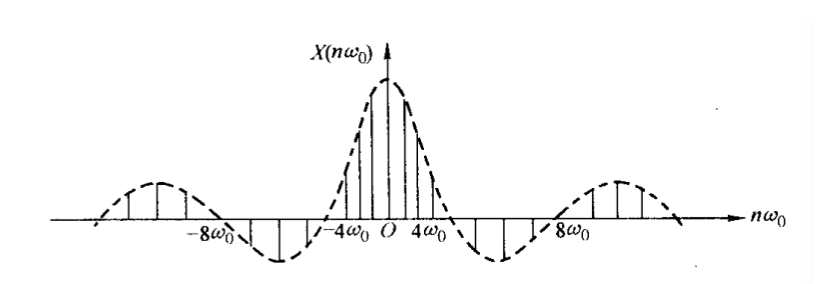由频谱图可以得出周期矩形脉冲信号的频谱具有三个特点：

（1）离散性：频谱是非周期性的离散的线状频谱，称它们为谱线，连接各谱线顶点的曲线为包络线，它反映了各频率分量的幅度随频率变化的情况。

（2）谐波性：谱线以基波频率 w 0 w_0 为间隔等距离分布，表明周期矩形脉冲信号只包含直流分量、基波分量和各次谐波分量。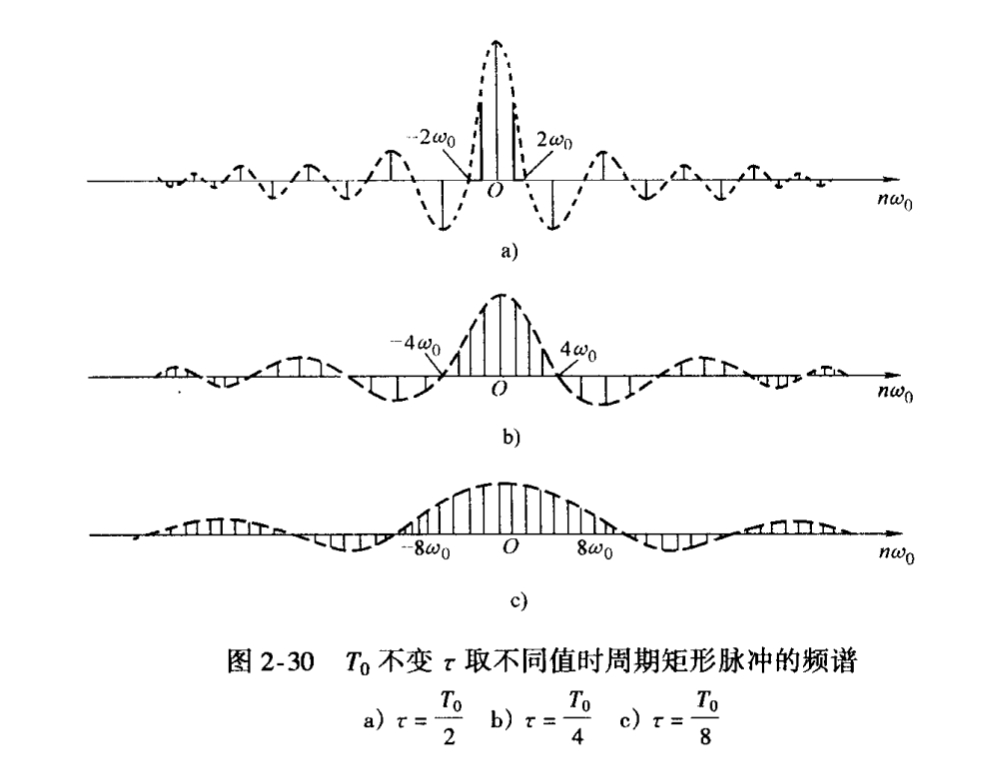T 0 T_0 不变而改变 τ \tau 不变而改变 τ \tau 从而使信号的占空比改变时，由于 w 0 w_0 不变，所以谱线之间的间隔不变，但随着 τ \tau 的减小（脉冲宽度减小），第一个过零点的频率增大，谱线的幅度减小。而将 τ \tau 固定，通过改变 T 0 T_0 来改变信号的占空比时，随着 T 0 T_0 增大，基波频率 w 0 w_0 减少，谱线将变得更密集，但第一个过零点的频率不变，谱线的幅度有所降低。作为极端情况，如果周期 T 0 T_0 无限增长，周期信号变成了非周期信号，这时，相邻谱线的间隔将趋于零，成为连续频谱。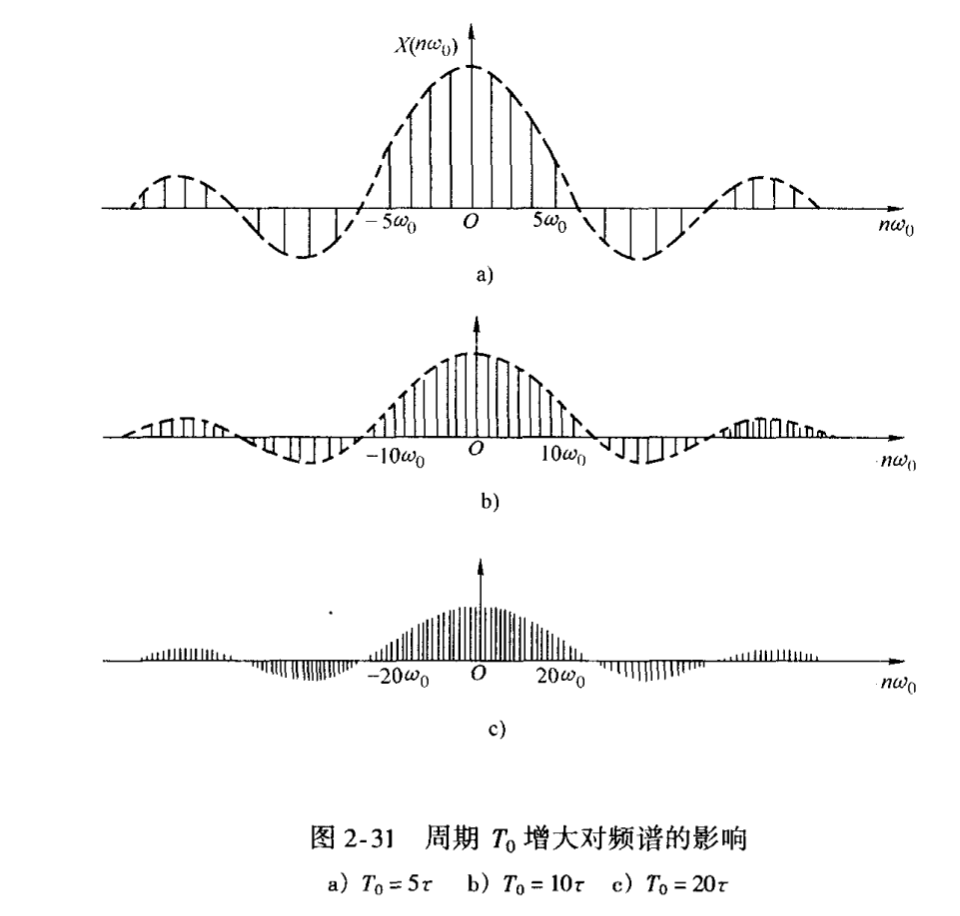（3）收敛性：谱线幅度整体上具有减小的趋势，同时，由于各谱线的幅度按包络线 S a ( 1 2 n w 0 τ ) Sa(\frac{1}{2}nw_0\tau) 的规律变化而等间隔地经过零点，较高幅值的谱线都集中在第一个过零点（ w = n w 0 = 2 π τ w=nw_0=\frac{2\pi}{\tau} ）范围内，表明信号的能量绝大部分由该频率范围的各谐波分量决定，通常把这个频率范围称为周期矩形脉冲信号的频带宽度或带宽，用符号 w b w_b f b f_b 表示。信号的带宽是信号频率特性中的重要指标，它具有实际意义。信号在其带宽内集中了大部分的能量，因此在允许一定失真的条件下，只需传送带宽内的各频率分量就行了；当信号通过某一系统时，要求系统的带宽与信号的带宽匹配，否则，若系统的带宽小于信号的带宽，信号中包含的一部分谐波分量和能量就不能顺利地通过系统。由上可知，脉冲宽度 τ \tau 越小，带宽 w b w_b 越大，频带内所含的分量越多。

以上三个特点时任何满足狄利赫里条件的周期信号的频谱所共同具有的。

例3：求出复指数信号 e j w 0 t e^{jw_0t} 的频谱。

：复指数信号 e j w 0 t e^{jw_0t} 的复傅里叶系数为
X ( n w 0 ) = 1 T 0 ∫ − T 0 2 T 0 2 e j w 0 t e j n w 0 t d t = 1 2 j ( 1 − n ) π [ e j ( 1 − n ) π − e − j ( 1 − n ) π ] = s i n ( 1 − n ) π ( 1 − n ) π = { 1 n = 1 0 n ≠ 1 X(nw_0)=\frac{1}{T_0}\int_{-\frac{T_0}{2}}^{\frac{T_0}{2}}e^{jw_0t}e^{jnw_0t}dt=\frac{1}{2j(1-n)\pi}[e^{j(1-n)\pi}-e^{-j(1-n)\pi}]\\ =\frac{sin(1-n)\pi}{(1-n)\pi}= \begin{cases} 1 & n=1 \\ 0 & n\neq 1 \end{cases}
可见仅在 w 0 w_0 处有幅度为1的分量，说明复指数信号是正弦信号的一种表现形式。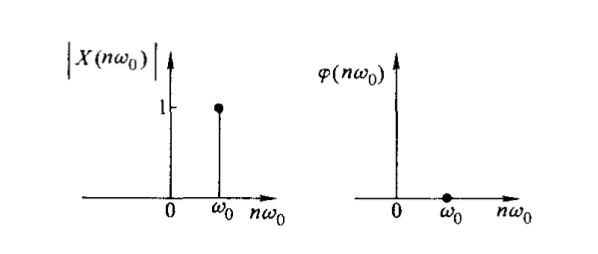例4：分别求出 c o s w 0 t cosw_0t s i n w 0 t sinw_0t 的频谱。

对于余弦信号 c o s w 0 t cosw_0t
X ( n w 0 ) = 1 T 0 ∫ − T 0 2 T 0 2 c o s w 0 t e − j n w 0 t d t = { 1 2 n = ± 1 0 n ≠ ± 1 X(nw_0)=\frac{1}{T_0}\int_{-\frac{T_0}{2}}^{\frac{T_0}{2}}cosw_0te^{-jnw_0t}dt= \begin{cases} \frac{1}{2} & n=\pm 1 \\ 0 & n\neq \pm 1 \end{cases}
对于正弦信号 s i n w 0 t sinw_0t
X ( n w 0 ) = 1 T 0 ∫ − T 0 2 T 0 2 s i n w 0 t e − j n w 0 t d t = { − j 2 n = 1 j 2 n = − 1 0 n ≠ ± 1 X(nw_0)=\frac{1}{T_0}\int_{-\frac{T_0}{2}}^{\frac{T_0}{2}}sinw_0te^{-jnw_0t}dt = \begin{cases} -\frac{j}{2} & n=1 \\ \frac{j}{2} & n=-1 \\ 0 & n\neq \pm1 \end{cases}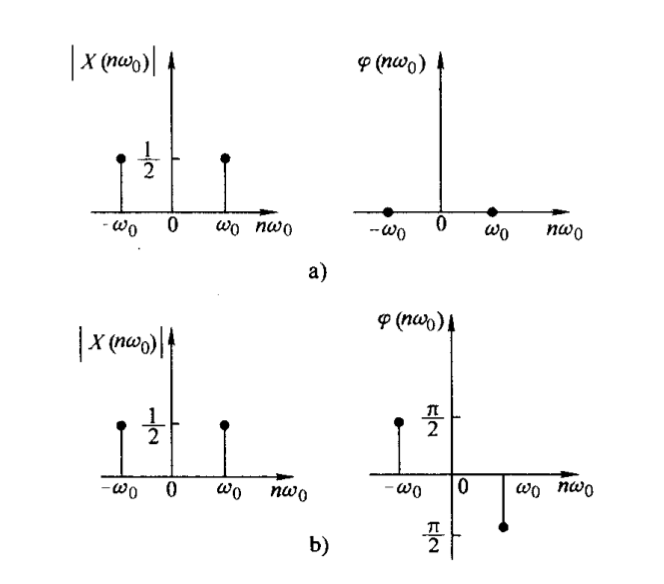它们的幅频相同。

负频率概念：频率作为周期信号变化快慢的一个度量，它只能是正值，即实际上只存在正频率，但在它的复指数形式的傅里叶级数表示法中会出现负频率，这只是数学上表示的需要，正是正、负频率的两个分量合起来（正负频率的幅度之和）才表示一个实际存在的正弦谐波分量。因此， c o s w 0 t cosw_0t s i n w 0 t sinw_0t 都是 w 0 w_0 处幅度为1的物理信号。此外， c o s w 0 t cosw_0t s i n w 0 t sinw_0t 的相频是不同的， s i n w 0 t sinw_0t 信号的相位滞后于 c o s w 0 t cosw_0t 信号的相位 π 2 \frac{\pi}{2}

展开全文• 周期信号可以看作为周期是无穷大的周期信号，从这一思想出发，可以在周期信号频谱分析的基础上研究非周期信号的频谱。在讨论矩形脉冲信号的频谱时，我们以及指出，当τ\tauτ不变而增大周期T0T_0T0​时，随着T0T_0...

### 非周期信号的频谱分析

非周期信号可以看作为周期是无穷大的周期信号，从这一思想出发，可以在周期信号频谱分析的基础上研究非周期信号的频谱。在讨论矩形脉冲信号的频谱时，我们以及指出，当 τ \tau 不变而增大周期 T 0 T_0 时，随着 T 0 T_0 的增大，谱线将越来越密，同时谱线的幅度将越来越小。如果 T 0 T_0 趋于无穷大时，则周期矩形脉冲信号将演变成非周期的矩形脉冲信号，可以预料，此时谱线会无限密集而演变成为连续的频谱，但与此同时，谱线的幅度将趋于零而变成无穷小量。为了避免在一系列无穷小量中讨论频谱关系，考虑 T 0 X ( n w 0 ) T_0X(nw_0) 这一物理量，由于 T 0 T_0 因子的存在，克服了 T 0 T_0 X ( n w 0 ) X(nw_0) 的影响。这时有 T 0 X ( n w 0 ) = 2 π X ( n w 0 ) w 0 T_0X(nw_0)=\frac{2\pi X(nw_0)}{w_0} ,即 T 0 X ( n w 0 ) T_0X(nw_0) 含有单位角频率所具有的复频谱的物理意义，故称为频谱密度函数，简称为频谱。

（一）从傅里叶级数到傅里叶变换

现在按上述思想建立非周期信号的频谱表示。考虑如下图所示的一个一般的非周期信号 x ( t ) x(t) ，它具有有限持续期，即 ∣ t ∣ > T 1 |t|>T_1 时， x ( t ) = 0 x(t)=0 。从这个非周期信号出发，构造一个周期信号 x ^ ( t ) \hat x(t) ，使 x ^ ( t ) \hat x(t) x ( t ) x(t) 进行周期为 T 0 T_0 的周期性延拓的结果。

[外链图片转存失败,源站可能有防盗链机制,建议将图片保存下来直接上传(img-4KtXD6uV-1592531737778)(/Users/can/Library/Application Support/typora-user-images/image-20200616113141271.png)]

对于周期信号 x ^ ( t ) \hat x(t) ，可以展开成指数形式的傅里叶级数
x ^ ( t ) = ∑ n = − ∞ ∞ X ^ ( n w 0 ) e j n w 0 t (1) \hat x(t)=\sum_{n=-\infty}^{\infty}\hat X(nw_0)e^{jnw_0t} \tag{1}
其中
X ^ ( n w 0 ) = 1 T 0 ∫ − T 0 2 T 0 2 x ^ ( t ) e − j n w 0 t d t \hat X(nw_0)=\frac{1}{T_0}\int_{-\frac{T_0}{2}}^{\frac{T_0}{2}}\hat x(t)e^{-jnw_0t}dt
考虑 T 0 X ^ ( n w 0 ) T_0\hat X(nw_0) ，并且由于在区间 − T 0 2 ≤ t ≤ T 0 2 -\frac{T_0}{2}\leq t\leq \frac{T_0}{2} x ^ ( t ) = x ( t ) \hat x(t)=x(t) ，则
T 0 X ^ ( n w 0 ) = ∫ − T 0 2 T 0 2 x ( t ) e − j n w 0 t d t (2) T_0\hat X(nw_0)=\int_{-\frac{T_0}{2}}^{\frac{T_0}{2}}x(t)e^{-jnw_0t}dt \tag{2}
T 0 → ∞ T_0\to \infty 时， x ^ ( t ) → x ( t ) , X ^ ( n w 0 ) → X ( n w 0 ) , w 0 → d w , n w 0 → w ( 连 续 量 ) \hat x(t)\to x(t),\hat X(nw_0)\to X(nw_0),w_0\to dw,nw_0\to w(连续量) T 0 X ( n w 0 ) T_0X(nw_0) 成为连续的频谱密度函数，记为 X ( w ) X(w) ，式（2）变为
X ( w ) = ∫ − ∞ ∞ x ( t ) e − j w t d t (3) X(w)=\int_{-\infty}^{\infty}x(t)e^{-jwt}dt \tag{3}
而式（1）变为
x ( t ) = l i m T 0 → ∞ ∑ n = − ∞ ∞ X ^ ( n w 0 ) e j n w 0 t = l i m T 0 → ∞ ∑ n = − ∞ ∞ T 0 X ^ ( n w 0 ) e j n w 0 t 1 T 0 = l i m T 0 → ∞ ∑ n = − ∞ ∞ 1 2 π T 0 X ^ ( n w 0 ) e j n w 0 t w 0 x(t)=lim_{T_0\to\infty}\sum_{n=-\infty}^{\infty}\hat X(nw_0)e^{jnw_0t}=lim_{T_0\to\infty}\sum_{n=-\infty}^{\infty}T_0\hat X(nw_0)e^{jnw_0t}\frac{1}{T_0} \\ =lim_{T_0\to\infty}\sum_{n=-\infty}^{\infty}\frac{1}{2\pi}T_0\hat X(nw_0)e^{jnw_0t}w_0
显然有
x ( t ) = 1 2 π ∫ − ∞ ∞ X ( ω ) e j w t d w (4) x(t)=\frac{1}{2\pi}\int_{-\infty}^{\infty}X(\omega)e^{jwt}dw \tag{4}
式（3）和式（4）构成了傅里叶变换对，通常表示成
F [ x ( t ) ] = X ( w ) F − 1 [ X ( w ) ] = x ( t ) F[x(t)] =X(w) \quad F^{-1}[X(w)]=x(t)
式（3）为傅里叶变换式，它将连续时间函数 x ( t ) x(t) 变换为频率的连续函数 X ( w ) X(w) ，因此 X ( w ) X(w) 称为 x ( t ) x(t) 的傅里叶变换。如前所述， X ( w ) X(w) 是频谱密度函数，为一复函数，即 X ( w ) = ∣ X ∣ e j φ ( w ) X(w)=|X|e^{j\varphi(w)} ，其模 ∣ X ( w ) ∣ |X(w)| 称为幅度频谱，幅角 φ ( w ) \varphi(w) 称为相位频谱，它在频域描述了信号的基本特征，因而是非周期信号进行频域分析的理论依据和最基本的公式。而式（4）为傅里叶反变换式，它把连续频率函数 X ( w ) X(w) 变换为连续时间函数 x ( t ) x(t) ，表明一个非周期信号是由频率为无限密集，幅度 X ( w ) ( d w 2 π ) X(w)(\frac{dw}{2\pi}) 等于无限小的无限多的复指数信号 e j w t e^{jwt} 线性组合而成。

式（4）也可以写成三角形式
x ( t ) = 1 2 π ∫ − ∞ ∞ X ( w ) e j w t d w = 1 2 π ∫ − ∞ ∞ ∣ X ( w ) ∣ e j [ w t + φ ( w ) ] d w = 1 2 π ∫ − ∞ ∞ ∣ X ( w ) ∣ c o s [ w t + φ ( w ) ] d w + j 2 π ∫ − ∞ ∞ ∣ X ( w ) ∣ s i n [ w t + φ ( w ) ] d w x(t)=\frac{1}{2\pi}\int_{-\infty}^{\infty}X(w)e^{jwt}dw=\frac{1}{2\pi}\int_{-\infty}^{\infty}|X(w)|e^{j[wt+\varphi(w)]}dw \\ =\frac{1}{2\pi}\int_{-\infty}^{\infty}|X(w)|cos[wt+\varphi(w)]dw+\frac{j}{2\pi}\int_{-\infty}^{\infty}|X(w)|sin[wt+\varphi(w)]dw
由于 ∣ X ( w ) ∣ |X(w)| 是w的偶函数， φ ( w ) \varphi(w) 是w的奇函数，故上式的第一个积分的被积函数是w的偶函数，第二个积分的被积函数是w的奇函数，因此有
x ( t ) = 1 π ∫ 0 ∞ ∣ X ( w ) ∣ c o s [ w t + φ ( w ) ] d w x(t)=\frac{1}{\pi}\int_0^{\infty}|X(w)|cos[wt+\varphi(w)]dw
表明一个非周期信号包含了频率从零到无限大的一切频率的余弦分量，而各分量的振幅 1 π ∣ X ( w ) ∣ d w \frac{1}{\pi}|X(w)|dw 是无穷小量，因此与周期信号不同，其频谱不能用幅度表示，而用频谱密度函数来表示， ∣ X ( w ) ∣ |X(w)| 可以看作为单位频率的振幅。

上面傅里叶变换的推导是由傅里叶级数演变来的，可以预料，一个函数 x ( t ) x(t) 的傅里叶变换是否存在应该看它是否满足狄利赫里条件，现在重新列出任意非周期函数 x ( t ) x(t) 存在傅里叶变换 X ( w ) X(w) 的狄利赫里条件如下：

1） x ( t ) x(t) 在无线区间内是绝对可积的，即
∫ − ∞ ∞ ∣ x ( t ) ∣ d t < ∞ \int_{-\infty}^{\infty}|x(t)|dt<\infty
2）在任意有限区间内， x ( t ) x(t) 只有有限个不连续点，在这些点上函数取有限值。

3）在任意有限区间内， x ( t ) x(t) 只有有限个极大值和极小值。

上述条件只是充分条件，倘若在变换中可以引入冲激函数或极限处理，那么在一个无限区间内不绝对可积的信号也可以认为具有傅里叶变换。

（二）常见非奇异信号的频谱

1. 矩形脉冲信号

g ( t ) = { E ∣ t ∣ < τ 2 0 ∣ t ∣ > τ 2 g(t)= \begin{cases} E & |t|<\frac{\tau}{2}\\ 0 & |t|>\frac{\tau}{2} \end{cases}

式中，E为脉冲幅度； τ \tau 为脉冲宽度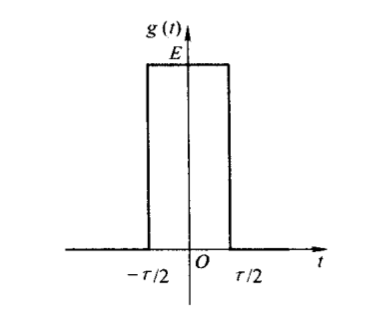其傅里叶变换为
X ( w ) = ∫ − ∞ ∞ g ( t ) e − j w t d t = ∫ − τ 2 τ 2 E e − j w t d t = E τ S a ( w τ 2 ) X(w)=\int_{-\infty}^{\infty}g(t)e^{-jwt}dt=\int_{-\frac{\tau}{2}}^{\frac{\tau}{2}}Ee^{-jwt}dt=E\tau Sa(\frac{w\tau}{2})
因为 X ( w ) X(w) 为一实函数，通常可用一条 X ( w ) X(w) 曲线同时表示幅度频谱和相位频谱，如图所示，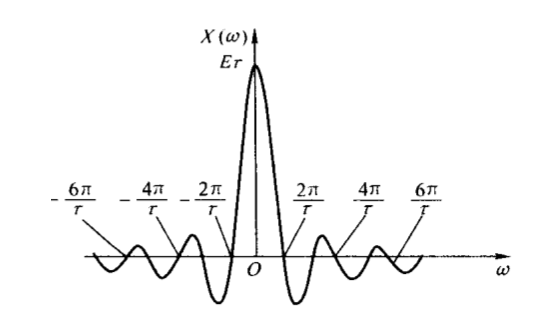与周期矩形脉冲的频谱图相比可以看出，单矩形脉冲的频谱 X ( w ) X(w) 与周期矩形脉冲频谱 X ( n w 0 ) X(nw_0) 的包络线形状完全相同，这正是由于将非周期的单矩形脉冲看作为周期是无穷大的周期矩形脉冲，从而其频谱由周期矩形脉冲的离散频谱演变为连续频谱的结果。另一方面， X ( w ) X(w) X ( n w 0 ) X(nw_0) 乘上因子 T 0 T_0 的结果，这是由于两者的不同定义决定的。

由于单脉冲信号与周期性脉冲信号的频谱存在上述联系，所以周期信号频谱的某些特点在单脉冲信号中仍有保留。单脉冲信号的频谱也具有收敛性，它的大部分能量集中在一个有限的频率范围内，常取从零频率到第一零值频率之间的频段为信号的频率宽度 w b w_b ，即 w b = 2 π τ w_b=\frac{2\pi}{\tau} .

显然，矩形脉冲越窄，它的频带宽度越宽。

1. 单边指数信号

x ( t ) = { e − a t t > 0 , a > 0 0 t < 0 x(t)= \begin{cases} e^{-at} & t>0,a>0 \\ 0 & t<0 \end{cases}

其傅里叶变换为
X ( w ) = ∫ − ∞ ∞ x ( t ) e − j w t d t = ∫ 0 ∞ e − a t e − j w t d t = 1 a + j w X(w)=\int_{-\infty}^{\infty}x(t)e^{-jwt}dt=\int_0^{\infty}e^{-at}e^{-jwt}dt=\frac{1}{a+jw}
幅频和相频分别为 ∣ X ( w ) ∣ = 1 a 2 + w 2 |X(w)|=\frac{1}{\sqrt{a^2+w^2}} φ ( w ) = − a r c t a n ( w a ) \varphi(w)=-arctan(\frac{w}{a})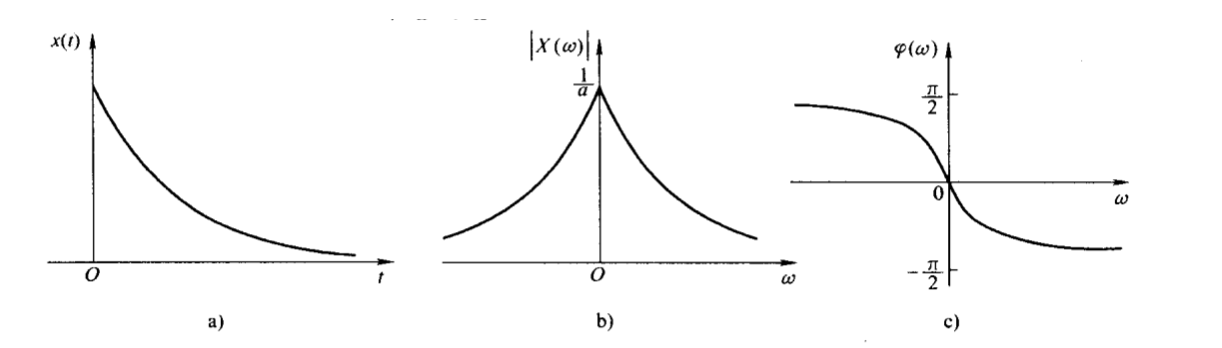1. 双边指数信号
x ( t ) = e − a ∣ t ∣ a > 0 x(t)=e^{-a|t|} \quad a>0

傅里叶变化为
X ( w ) = ∫ − ∞ ∞ e − a ∣ t ∣ e − j w t d t = ∫ − ∞ 0 e − a t e − j w t d t + ∫ 0 ∞ e a t e − j w t d t = 2 a a 2 + w 2 X(w)=\int_{-\infty}^{\infty}e^{-a|t|}e^{-jwt}dt=\int_{-\infty}^0e^{-at}e^{-jwt}dt+\int_0^{\infty}e^{at}e^{-jwt}dt = \frac{2a}{a^2+w^2}
X ( w ) X(w) 是实数， φ ( w ) = 0 \varphi(w)=0 ，如下图。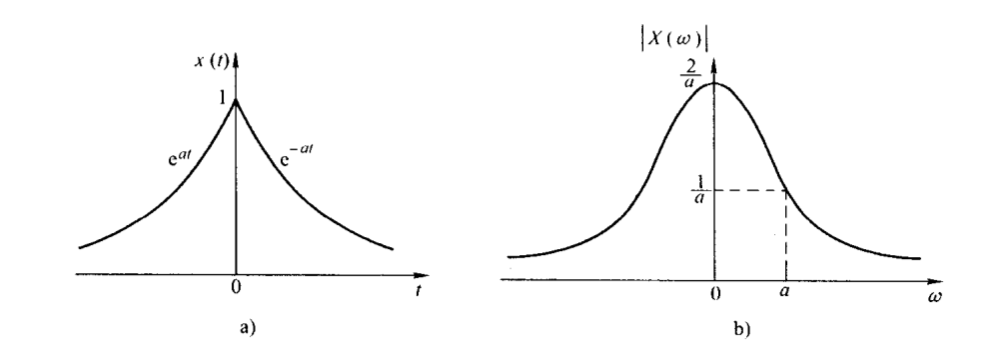1. 双边奇指数信号及其频谱

x ( t ) = { − e a t t < 0 , a > 0 e − a t t > 0 , a > 0 x(t)= \begin{cases} -e^{at} & t<0,a>0 \\ e^{-at} & t>0,a>0 \end{cases}

其傅里叶变换
X ( w ) = ∫ − ∞ ∞ x ( t ) e − j w t d t = ∫ − ∞ 0 ( − e a t e − j w t ) d t + ∫ 0 ∞ e − a t e − j w t d t = − j 2 w a 2 + w 2 X(w)=\int_{-\infty}^{\infty}x(t)e^{-jwt}dt=\int_{-\infty}^0(-e^{at}e^{-jwt})dt+\int_0^{\infty}e^{-at}e^{-jwt}dt = -j\frac{2w}{a^2+w^2}
其幅频和相频分别为
∣ X ( w ) ∣ = 2 ∣ w ∣ a 2 + w 2 |X(w)|=\frac{2|w|}{a^2+w^2}

φ ( w ) = { π 2 w < 0 − π 2 w > 0 \varphi(w)= \begin{cases} \frac{\pi}{2} & w<0 \\ -\frac{\pi}{2} & w>0 \end{cases}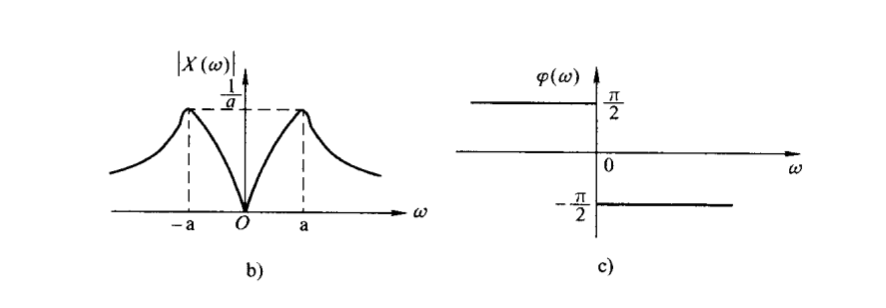展开全文• 由此式可得知，该信号频谱谱线大致按照采样函数（Sa(t)）形状分布。 频谱如下图所示： 观察该谱线可得如下特点： 频谱为离散谱线 谱线幅度以 Sa(kω0τ/2) 为包络线变化 在 ω = 2mπ/τ 处过零点 主频带宽度为： ...
• 一、周期信号频谱 1.1周期信号的虚指数/三角傅里叶级数： 其中， 1.2 意义：从频域上看，周期信号可以由其所有频率分量叠加而成，并且这些频域分量都是周期信号频率的整数倍，傅里叶级数的作用就是算出这些...matlab
• 由此式可得知，该信号频谱谱线大致按照采样函数（Sa(t)）形状分布。 谱线及特点 周期矩形脉冲信号的频谱由下图所示： 观察该谱线可得如下特点： 频谱为离散谱线 谱线幅度以 Sa(kω0τ/2) 为包络线变化 在 ω = 2mπ...
• 通过应用结果，推导了离散频谱连续频谱之间的关系，并提出了一种从一维情况下的周期性非均匀采样信号重构数字频谱的方法。 然后，我们将该理论扩展到二维情况。 最后，仿真结果表明了该方法的优势和有效性。
• 周期信号频谱 之前我们已经了解到了，任一满足狄利克雷条件的周期信号都可以进行傅里叶级数展开；分解成直流分量以及许多正余弦分量。 这些正余弦分量的频率必定是基频(基波频率)的整数倍，响应的频率分量分别为...
• 在研究周期性振动信号处理方法的基础上,针对连续非周期信号难以有效识别的问题,提出了一种基于小波变换和自功率谱相结合的故障诊断方法,采用小波变换对源信号进行去噪处理,并对处理后的信号进行自功率谱分析,解决了...
• 在学习傅里叶变换的时候遇到了求周期方波信号频谱图的例子，在书上和网上查阅了一些资料，发现大都是讨论的都是下图左边的周期信号的频谱，课程老师的PPT中也只列出了另一种周期信号频谱图的结论，没有在进行傅里叶...
• （三）周期信号的功率分配 幅度有限的周期信号是功率信号，如果把信号x(t)x(t)x(t)视为加在1Ω1\Omega1Ω电阻两端的电压或通过的电流，那么电阻上消耗的平均功率为 p=1T0∫−T02T02x2(t)dt p=\frac{1}{T_0}\int_{-\...
• 1.3 扩展分析：当周期信号周期T很大的情况下的频谱 二、非周期矩形信号频谱【由x(t)求X(f)】 三、如何通过频谱X(f)求信号x(t) 四、傅里叶变换 一、周期方波的频谱分析【由x(t)求X(f)】 1.1 周期方波的复...
• 基于MATLAB仿真的连续信号频谱分析.doc.doc实验十：基于MATLAB仿真的连续信号频谱分析一、实验目的1、学会运用MATLAB求连续时间信号的傅里叶变换；2、学会运用MATLAB求连续时间信号的频谱图。二、实验原理1、周期...
• 文章目录周期信号的频谱及特点1 周期信号的频谱2 单边谱和双边谱的关系3 周期信号频谱的特点4 周期信号的功率 周期信号的频谱及特点 频谱——信号的一种新的表示方法 1 周期信号的频谱 频谱：周期信号分解后，各...
• 7 时域、周期性连续信号的傅里叶级数 7.1周期性、连续信号的傅里叶级数概述 时域上任意连续的、周期信号可以分解为无限多个、离散的、非周期的、正交的复指数信号之和，称之为傅里叶级数。 （1）时域信号...常见信号的频谱
• 周期信号频谱 傅里叶系数的幅度|Fn|或者An随角频率nw0变化的规律称为信号的幅度频谱，简称幅度谱；傅里叶系数的相位Φn随角频率nw0变化的规律称为信号的相位频谱，简称相位谱。 三角频谱（单边频谱） 三角频谱...
• 这是重点 单位频率上的频谱 傅里叶变换 这和之前的频谱不一样 ...
• 对于一个离散时间周期信号x(n)x(n)x(n)，可以通过IDFS从它的周期性离散频谱X(kΩ0)X(k\Omega_0)X(kΩ0​)求得原始序列x(n)x(n)x(n)，它们是一一对应的关系。也就是说，用有限项的复指数序列来表示周期序列x(n)x(n)x...
• 例：为了说明时域加窗对连续信号频谱分析的影响，现分析一无穷长的余弦信号的频谱。 加窗 抽样 DFT 加窗 抽样 DFT 结 论 加窗处理对信号频谱分析影响： 加窗后的频谱存在更多高频成份。 谱线变成了具有一定宽度的谱...
• 第3章利用DFT做连续信号频谱分析3.2 利用DFT做连续信号的频谱分析 3.2 利用DFT做连续信号的频谱分析 信号的频谱分析：利用DFT计算连续信号的频谱 3.2 利用DFT做连续信号的频谱分析 信号的频谱分析：利用DFT计算连续...
• 它们的特点是在一个周期内的极值点不会超过两个且周期性特征明显。对于这类已明确具有周期特性的信号，周期与否的判别相对简单，周期测量的方法也很成熟完善，如：过零检测法，脉冲整形法等。周期信号表达式x(t)=x(t...
• 首先回顾一下在信号与系统（10）-周期性信号频谱中提及的方波脉冲信号，如果脉冲宽度τ\tauτ进行无线增大，则信号变为非周期信号，并且幅度频谱由离散谱变为连续频谱，如下所示： 周期性方波脉冲，即： f(t)={A,&...
• 首先对信号进行 scipy 中的 fft 变换，得到和原始信号相同长度的变换结果 fft_y, 这个结果包含振幅和相位信息 变换得到的结果包含相位谱和振幅谱，通过 abs(fft_y) 可以直接得到变换后结果的"双边"振幅谱；通过np....python
• 学 海 无 涯 非周期信号的傅里叶变换MATLAB 仿真实验 一实验目的 1熟悉连续周期信号频谱特点及其分析方法 2 掌握用MATLAB 实现傅里叶变换 二非周期信号的傅里叶变换原理及性质 设周期信号 f (t) 展开成复指数形式...
• 一、实验目的：1、掌握傅立叶级数(FS)，学会分析连续时间周期信号频谱分析及MATLAB实现；2、掌握傅立叶变换(FT)，了解傅立叶变换的性质以及MATLAB实现。二、利用符号运算求傅里叶级数的系数1、复习几个函数：F1=...
• 周期矩形脉冲信号频谱分析(2) 互相关函数R12(τ)=R21(-τ)。 (3) 自相关函数R(τ)=R(-τ)是偶函数。 (4) 自相关函数R(0)的物理意义是：对于能量信号 对功率信号 (5) R(0)≥R(τ)。 2.6.2 归一化相关函数和相关系数...
• 一，实验名称： DFT 的频谱分析 二，实验目的：1. 加深对 DFT 原理的理解，熟悉DFT 的性质。2. 掌握离散傅里叶变换的有关...连续信号与系统的傅里叶分析显然不便于直接用计算机进行计算，使其应用受到限制，而DFT ...
• matlaB函数连续周期三角波信号频谱图N=10;n1=-N:-1;C1=-4*j*sin(n1*pi/2)/pi^2./n1.^2;C0=0;n2=1:N;C2=-4*j*sin(n2*pi/2)/pi^2./n2.^2;Cn=[C1 C0 C2];n=-N:N;subplot(2,1,1);stem(n,abs(Cn));subplot(2,1,2);stem(n,...
• 第七章 信号频域分析及 MATLAB 实现 7.1 周期信号的傅利叶级数与信号频谱 7.2 周期信号频谱分析及MATLAB实现 7.3 用MATLAB分析典型周期信号频谱 7.1 周期信号的傅里叶级数与信号频谱 7.1 周期信号的傅里叶......